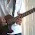## Method to Control 8*8 LED Matrix using Shift Register IC 74595 and Arduino Mega

by realfinetime  |  in LED Matrix at  22:05

In the past few blogs, we found the basics of working of 8*8 LED matrix and shift register IC 74595. In this blog, we will see the circuit to connect 8*8 LED matrix to arduino through shift registers using minimum number of digital pins of arduino. Essence of this circuit is, controlling 64 LEDs using only six digital output pins from arduino. Circuit is given at the bottom of this page. Before starting the circuit, you should be familiar about the pinout diagram of, 8*8 LED matrix and 74595 shift register IC.

Pinout diagram of 8*8 LED matrix

Pinout diagram of 8*8 LED matrix is given below. Refer this blog to get a simple circuit to demonstrate the working of 8*8 LED matrix. Small wedges on the middle of four edges of the 8*8 LED matrix is the indication of the pin from which pin numbering should be started.
Internal Circuit of 8*8 LED matrix

Internal circuit of 8*8 LED matrix is given below. Pin numbering of 8*8 LED matrix is a bit confusing. Read this blog to get a clear idea about the pin numbering of 8*8 LED matrix.

Pin out diagram of 74595

Pinout diagram of 74595 is given below. Two 74595 ICs are used in this circuit. Read this blog to get an idea about the working of 74595.

Next is the circuit diagram. Two 74595 ICs are used to control one LED matrix. One IC is for controlling the rows of LED matrix and the other IC is for controlling the columns of LED matrix. 5V for the working of IC is given from a 5V regulator. SHCP, STCP and DS pins of both 74595 are connected to separate digital output pins of arduino as shown in the circuit. VCC and MRbar of both ICs should be connected to 5V. Gnd and OEbar of both ICs should be connected to the Gnd of voltage source. 100 Ohm current limiting resistors should be connected to the cathode pins of 8*8 LED matrix. If current limiting resistor is connected to the anode pins of 8*8 LED matrix, brightness of LEDs will decrease, when more LEDs will turn on. Gnd pin of arduino should be connected to the gnd pin of voltage source for voltage leveling.

Connection between arduino mega and 1st 74595 can be summarized as follows.

1.  Digital pin 11 of arduino is connected to the DS pin (14th pin) of 1st 74595.
2.  Digital pin 12 of arduino is connected to the STCP pin (12th pin) of 1st 74595.
3.  Digital pin 13 of arduino is connected to the SHCP pin (11th pin) of 1st 74595.

Connection between arduino mega and 2nd 74595 can be summarized as follows.

1.  Digital pin 5 of arduino is connected to the DS pin (14th pin) of 2nd 74595.
2.  Digital pin 6 of arduino is connected to the STCP pin (12th pin) of 2nd 74595.
3.  Digital pin 7 of arduino is connected to the SHCP pin (11th pin) of 2nd 74595.

Connection between 1st 74595 and 8*8 LED matrix can be summarized as follows.

1.  Q0 pin (15th pin) of 1st 74595 is connected to the 16th pin 8*8 LED matrix.
2.  Q1 pin (1st pin) of 1st 74595 is connected to the 15th pin 8*8 LED matrix.
3.  Q2 pin (2nd pin) of 1st 74595 is connected to the 11th pin 8*8 LED matrix.
4.  Q3 pin (3rd pin) of 1st 74595 is connected to the 3rd pin 8*8 LED matrix.
5.  Q4 pin (4th pin) of 1st 74595 is connected to the 10th pin 8*8 LED matrix.
6.  Q5 pin (5th pin) of 1st 74595 is connected to the 5th pin 8*8 LED matrix.
7.  Q6 pin (6th pin) of 1st 74595 is connected to the 6th pin 8*8 LED matrix.
8.  Q7 pin (7th pin) of 1st 74595 is connected to the 13th pin 8*8 LED matrix.

Connection between 2nd 74595 and 8*8 LED matrix can be summarized as follows.

1.  Q0 pin (15th pin) of 2nd 74595 is connected to the 4th pin 8*8 LED matrix.
2.  Q1 pin (1st pin) of 2nd 74595 is connected to the 7th pin 8*8 LED matrix.
3.  Q2 pin (2nd pin) of 2nd 74595 is connected to the 2nd pin 8*8 LED matrix.
4.  Q3 pin (3rd pin) of 2nd 74595 is connected to the 8th pin 8*8 LED matrix.
5.  Q4 pin (4th pin) of 2nd 74595 is connected to the 12th pin 8*8 LED matrix.
6.  Q5 pin (5th pin) of 2nd 74595 is connected to the 1st pin 8*8 LED matrix.
7.  Q6 pin (6th pin) of 2nd 74595 is connected to the 14th pin 8*8 LED matrix.
8.  Q7 pin (7th pin) of 2nd 74595 is connected to the 9th pin 8*8 LED matrix.

1.Is it possible to use similar circuit to drive an 8x8 stepper-motors matrix?

1.of course but must be controlled by only, cannot apply directo to motors, it can burn the arduino board

2.Is it possible to use similar circuit to drive an 8x8 stepper-motors matrix?

3.Excellent guide, sincere congratulations

4.Hi, my name is marlin. Good article, this is very interesting and can increase my knowledge. thank you for sharing. always success

Arduino Tutorial For Begginers

Arduino Uno Project For Beginners

How To Reset Arduino Uno

5.Great Information. A debt of gratitude is in order for sharing valuable data.
led screen rental

6.Nice information. You will get something to learn new. Visit Led Video Screen For Sale to browse outdoor and indoor LED video wall prices.

## IMPORTANT NOTICE

All the circuits, published in this blog is only after testing and getting proper results in my private lab. When you try these circuits, you should check the supply voltage, polarity of components, presence of childrens nearby and shorts in the circuits. This website will not be responsible for any harm happened to you or your components caused by your carelessness.